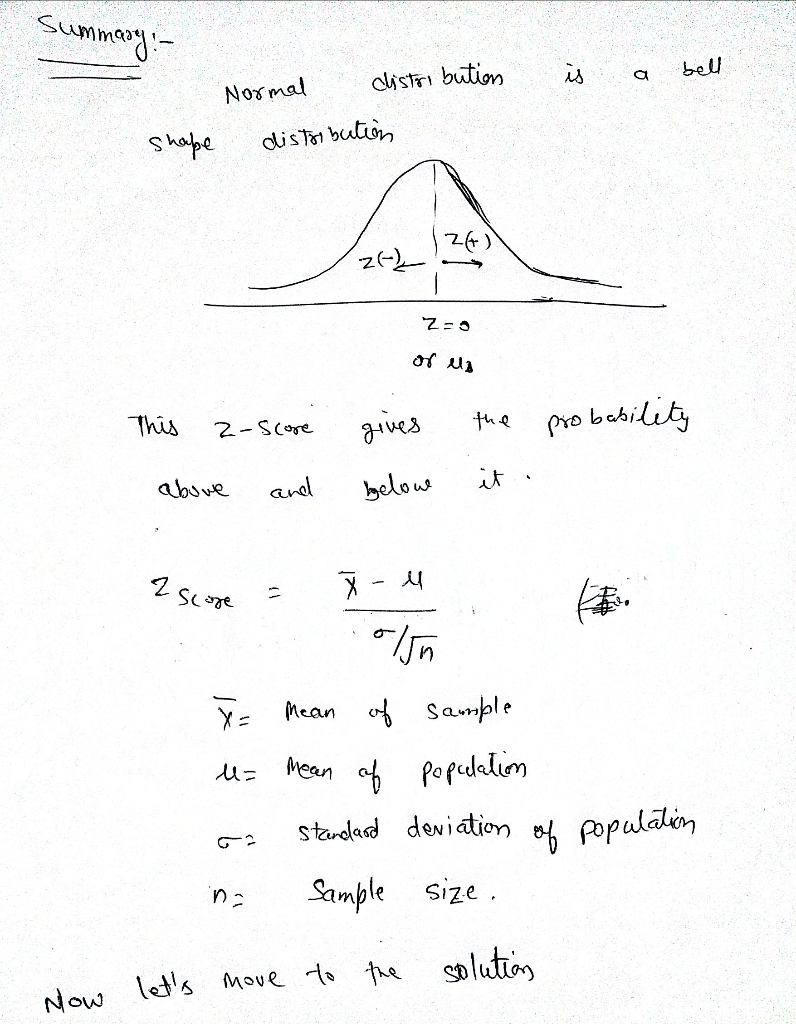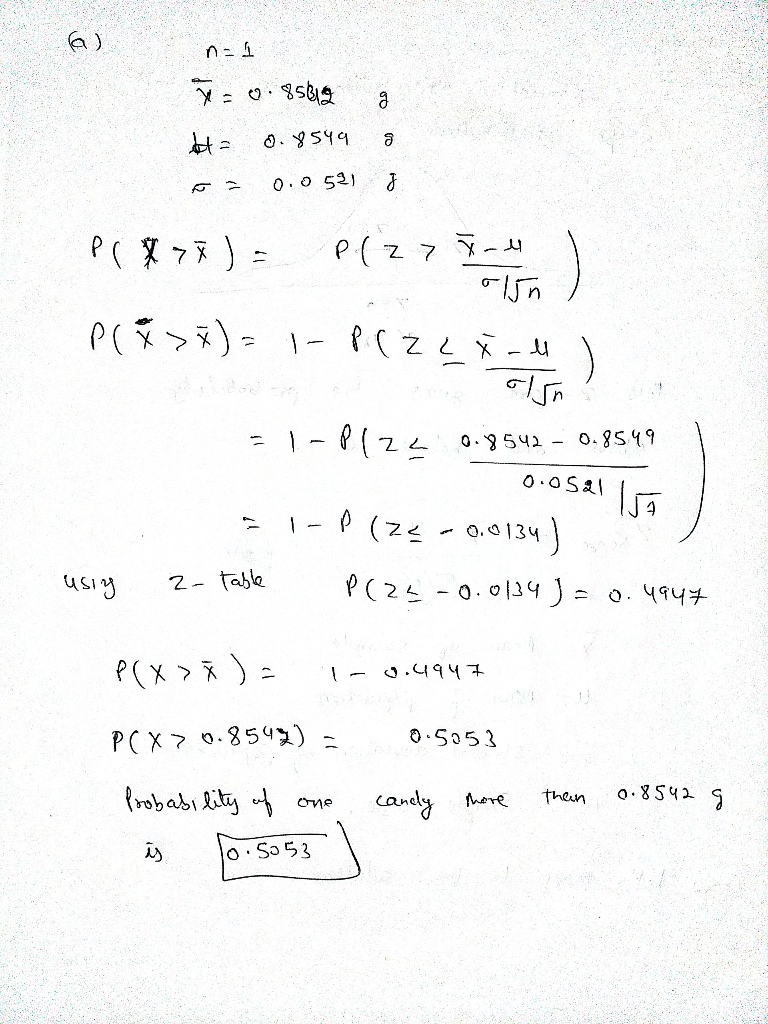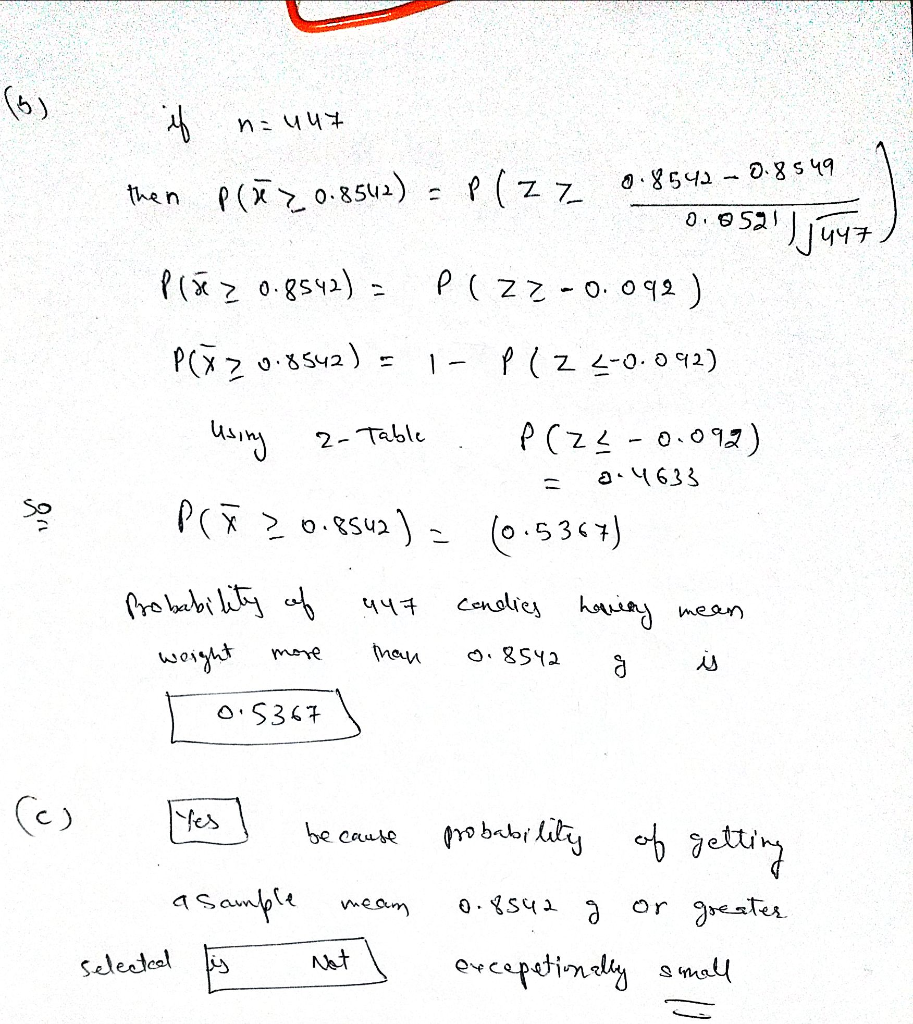##### Question

In: Statistics and Probability

# The weights of a certain brand of candies are normally distributed with a mean weight of...

The weights of a certain brand of candies are normally distributed with a mean weight of 0.8549 g and a standard deviation of 0.0521 g. A sample of these candies came from a package containing 456​candies, and the package label stated that the net weight is 389.2 g.​ If every package has 456 candies, the mean weight of the candies must exceed 389.2/456 = 0.8536 for the net contents to weigh at least 389.2 ​g. a) if 1 candy is randomly selected, find the probability that it weights more than 0.8542g. the probability is.... b) If 447 candies are reandomly selected find the probability that their mean weight is at least 0.8542 g. the probability that a sample of 447 candies will have a mean of 0.8542g or greater is __ (round to four decimal places as needed) c) given these results does it seem that the candy company is providing consumers with the amount claimed on the label?

## Solutions

##### Expert Solution## Related Solutions

##### The weights of a certain brand of candies are normally distributed with a mean weight of...
The weights of a certain brand of candies are normally distributed with a mean weight of 0.8541 g and a standard deviation of 0.0517g. A sample of these candies came from a package containing 440 candies and the package label stated that the net weight is 375.6 ( If every package has 440 candies, the mean weight of the candies must exceed 374 / 440= 0.8536 g for the net contents to weigh at least 375.6 g)g.) a. If 1...
##### The weights of a certain brand of candies are normally distributed with a mean weight of...
The weights of a certain brand of candies are normally distributed with a mean weight of 0.85860.8586 g and a standard deviation of 0.05170.0517 g. A sample of these candies came from a package containing 464464 ​candies, and the package label stated that the net weight is 396.0396.0 g.​ (If every package has 464464 ​candies, the mean weight of the candies must exceed StartFraction 396.0 Over 464 EndFraction396.0464equals=0.85340.8534 g for the net contents to weigh at least 396.0396.0 ​g.) a....
##### The weights of a certain brand of candies are normally distributed with a mean weight of...
The weights of a certain brand of candies are normally distributed with a mean weight of 0.8541 g and a standard deviation of 0.0516 g. A sample of these candies came from a package containing 440 ​candies, and the package label stated that the net weight is 375.5 g.​ (If every package has 440 ​candies, the mean weight of the candies must exceed 375.5/440 =0.8534 g for the net contents to weigh at least 375.5 g.) a. If 1 candy...
##### The weights of a certain brand of candies are normally distributed with a mean weight of...
The weights of a certain brand of candies are normally distributed with a mean weight of 0.85550.8555 g and a standard deviation of 0.05170.0517 g. A sample of these candies came from a package containing 458458 ​candies, and the package label stated that the net weight is 391.4391.4 g.​ (If every package has 458458 ​candies, the mean weight of the candies must exceed StartFraction 391.4 Over 458 EndFraction391.4458equals=0.85450.8545 g for the net contents to weigh at least 391.4391.4 ​g.) a....
##### The weights of a certain brand of candies are normally distributed with a mean weight of...
The weights of a certain brand of candies are normally distributed with a mean weight of 0.8617 g and a standard deviation of 0.0517 g. A sample of these candies came from a package containing 440 ​candies, and the package label stated that the net weight is 375.5 g.​ (If every package has 440 ​candies, the mean weight of the candies must exceed StartFraction 375.5 Over 440 EndFraction equals0.8535 g for the net contents to weigh at least 375.5 ​g.)
##### The weights of a certain brand of cereal boxes are normally distributed. The mean weight of...
The weights of a certain brand of cereal boxes are normally distributed. The mean weight of a SAMPLE of 13 boxes was 14.91 ounces with a sample standard deviation of .22 ounces. The margin of error on a 90% confidence interval would be smaller than the margin of error on a 95% confidence interval. True or false?
##### The weights of the fish in a certain lake are normally distributed with a mean of...
The weights of the fish in a certain lake are normally distributed with a mean of 20 lb and a standard deviation of 9. If 9 fish are randomly selected, what is the probability that the mean weight will be between 17.6 and 23.6 lb? Write your answer as a decimal rounded to 4 places.
##### The weights of the fish in a certain lake are normally distributed with a mean of...
The weights of the fish in a certain lake are normally distributed with a mean of 9.4 lb and a standard deviation of 2.3. If 42 fish are randomly selected, what is the probability that the mean weight will be more than 9.7 lb?
##### The weights of the fish in a certain lake are normally distributed with a mean of...
The weights of the fish in a certain lake are normally distributed with a mean of 13 pounds and a standard deviation of 6. If a sample of 9 fish are randomly selected, what is the probability that the mean weight will be between 10.2 and 16.6 pounds?
##### The weights of the fish in a certain lake are normally distributed with a mean of...
The weights of the fish in a certain lake are normally distributed with a mean of 9.9 lb and a standard deviation of 2.1. If 75 fish are randomly selected, what is the probability that the mean weight will be between 7.7 and 10.4 lb?